# Selina Solutions Concise Maths Class 10 Chapter 3 Shares and Dividends

Firstly, the term share is defined and then some basic terms like nominal value (N.V), market value (M.V), at a premium, at a discount and dividend are discussed in this chapter. Students who are willing to clear their doubts and improve their problem-solving skills can make use of Selina Solutions. This is a very useful resource for students to prepare confidently for their examinations. All these solutions are prepared by subject experts at BYJU’S on par with the latest examination patterns.

Chapter 3 of Concise Selina for Class 10 Maths has three exercises. Problems based on finding the profit or loss (percentage), dividend, number of shares, annual income are included in this chapter. Students can now access the Concise Selina Solutions for Class 10 Maths Chapter 3 Shares and Dividends PDF from the links given below.

## Selina Solutions Concise Maths Class 10 Chapter 3 Shares and Dividends Download PDF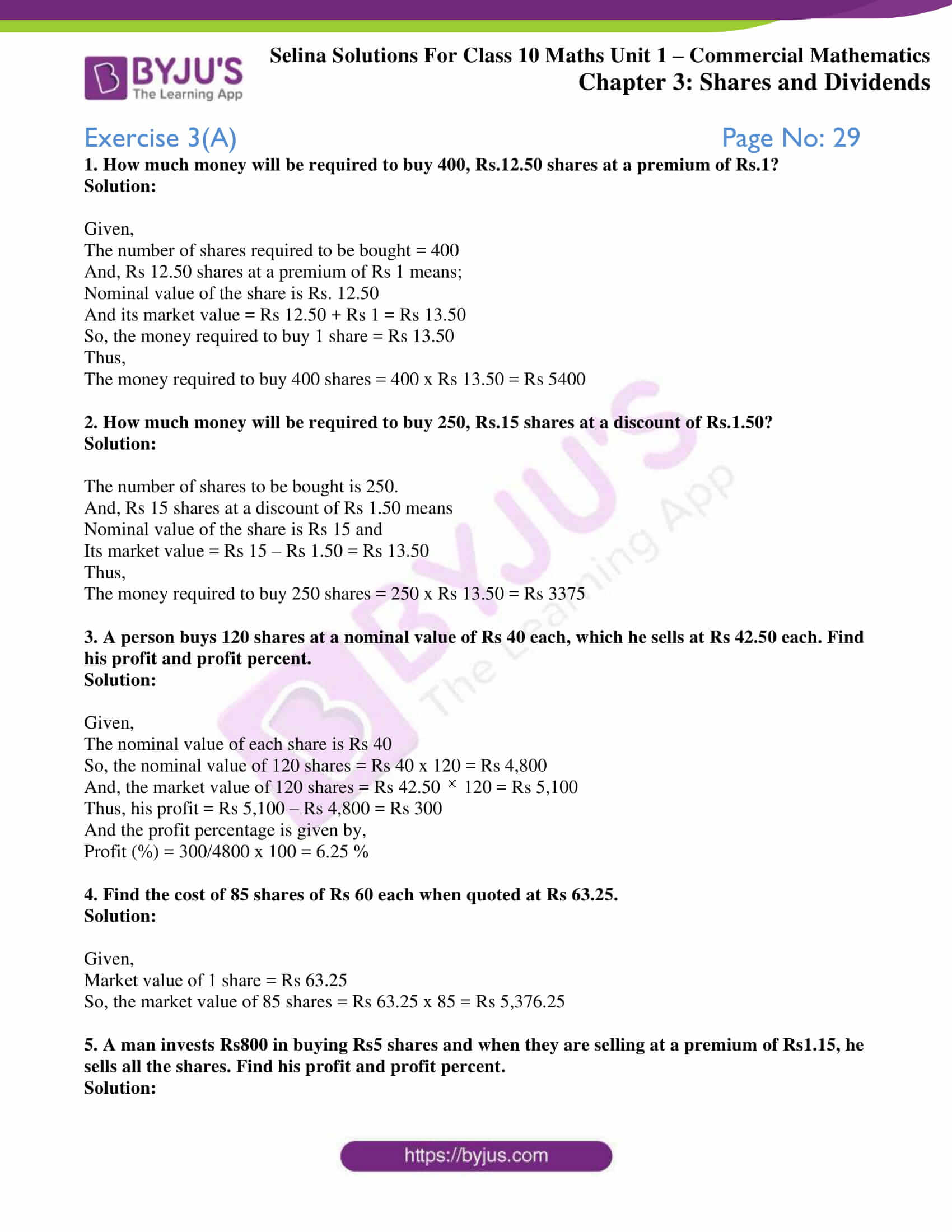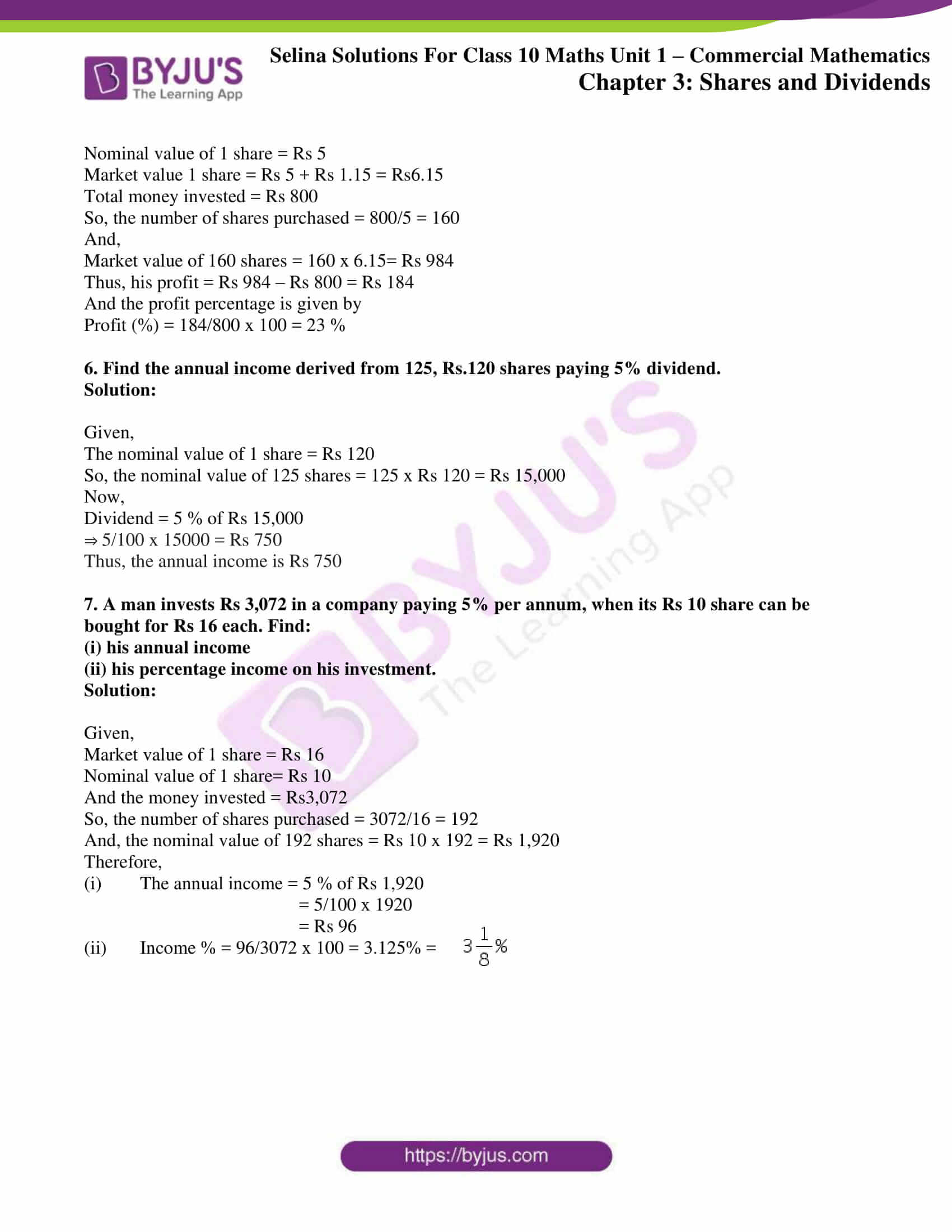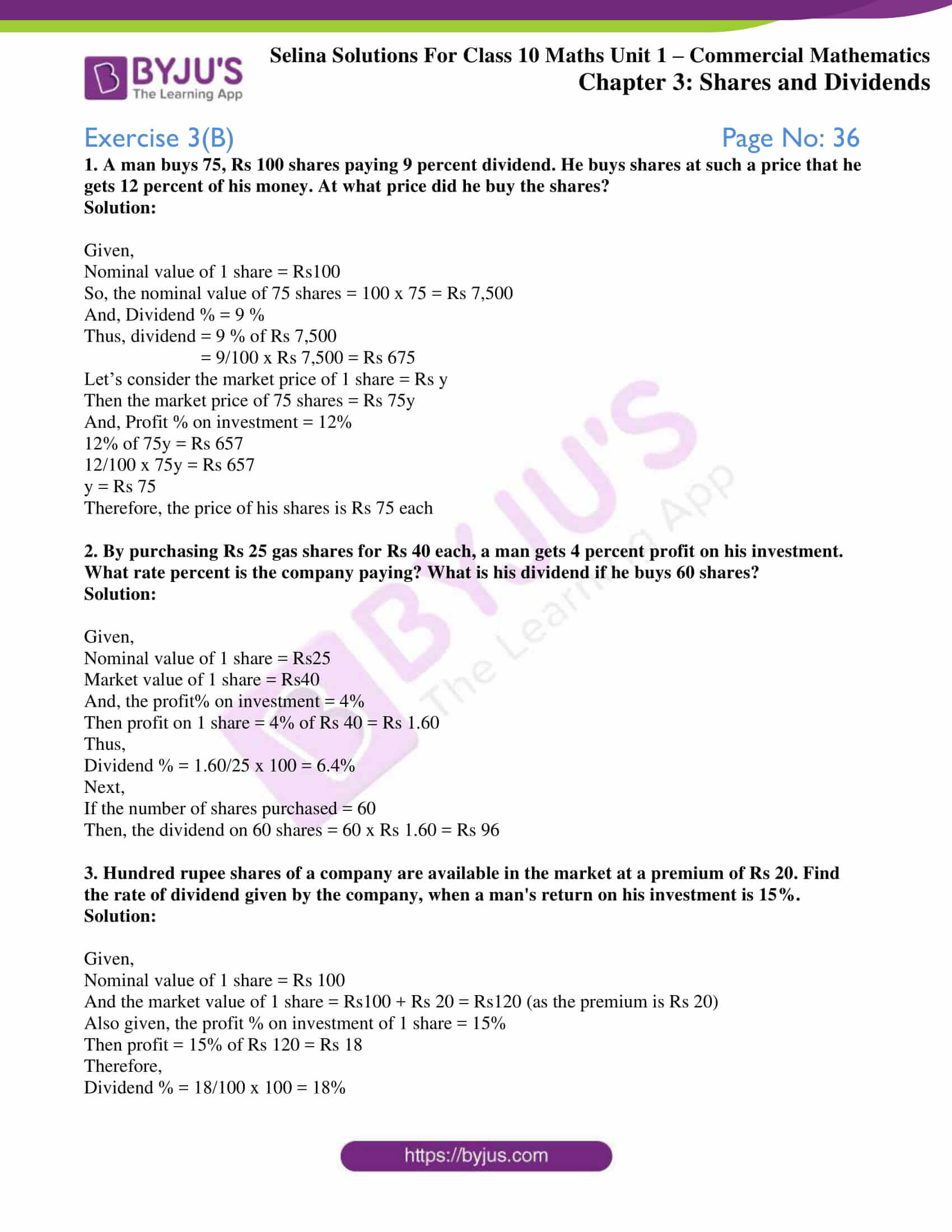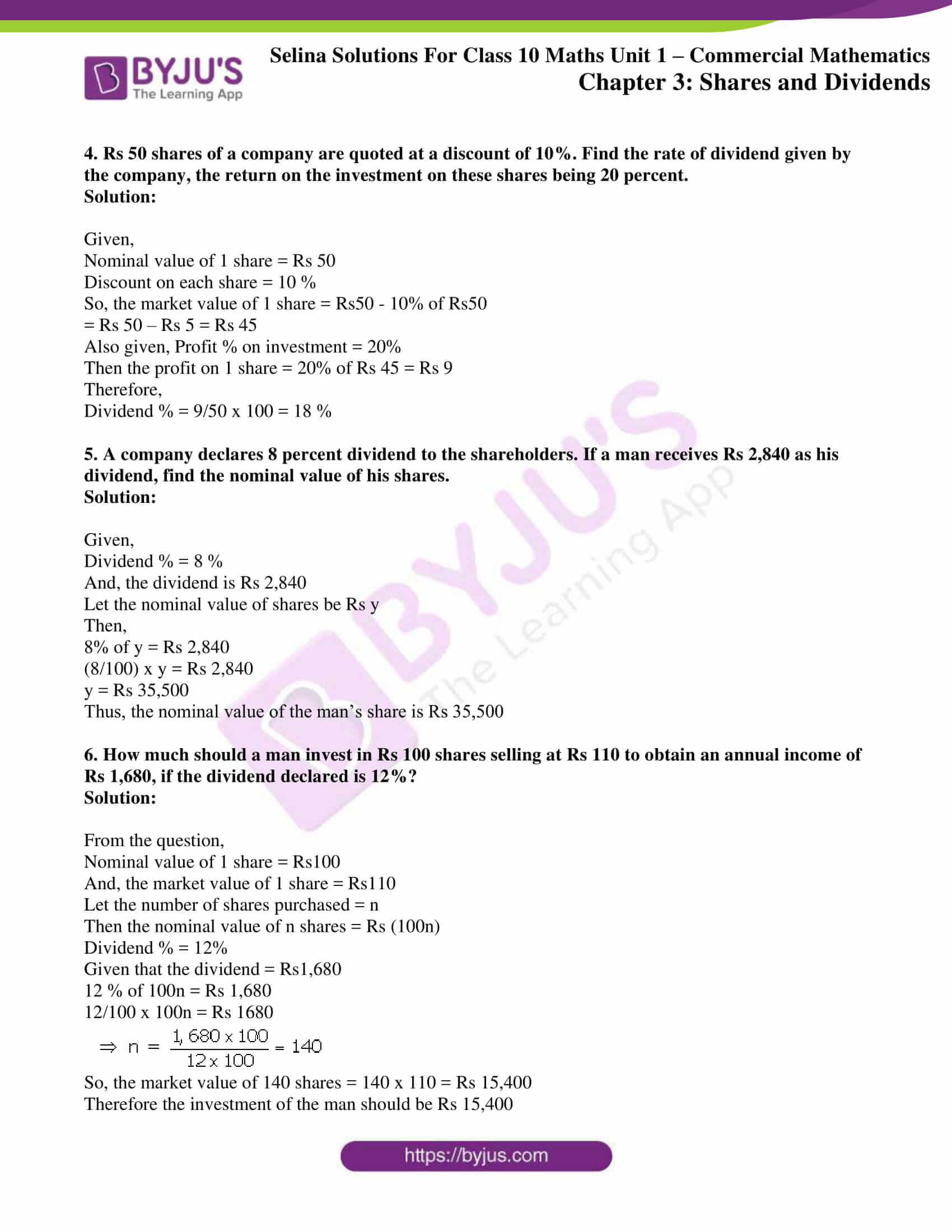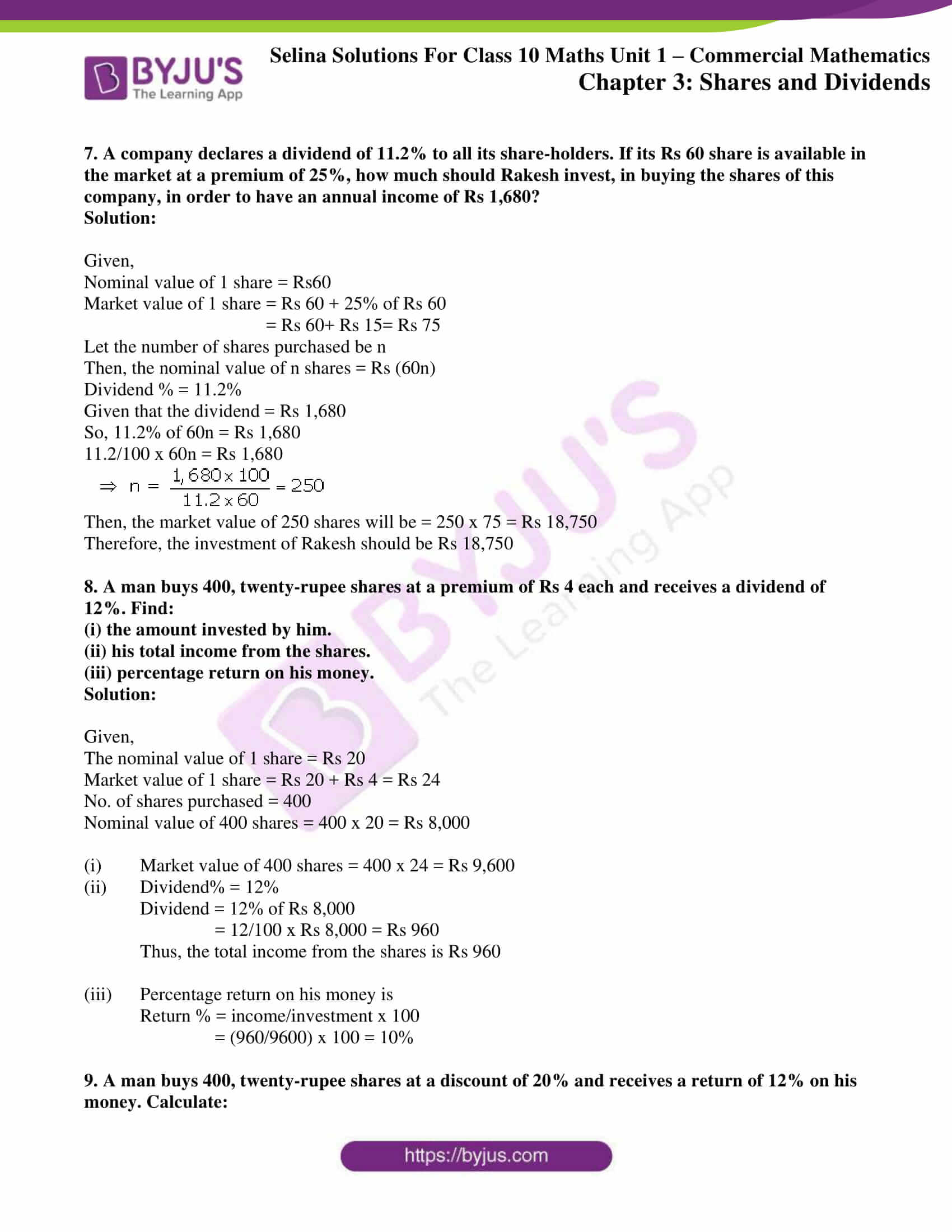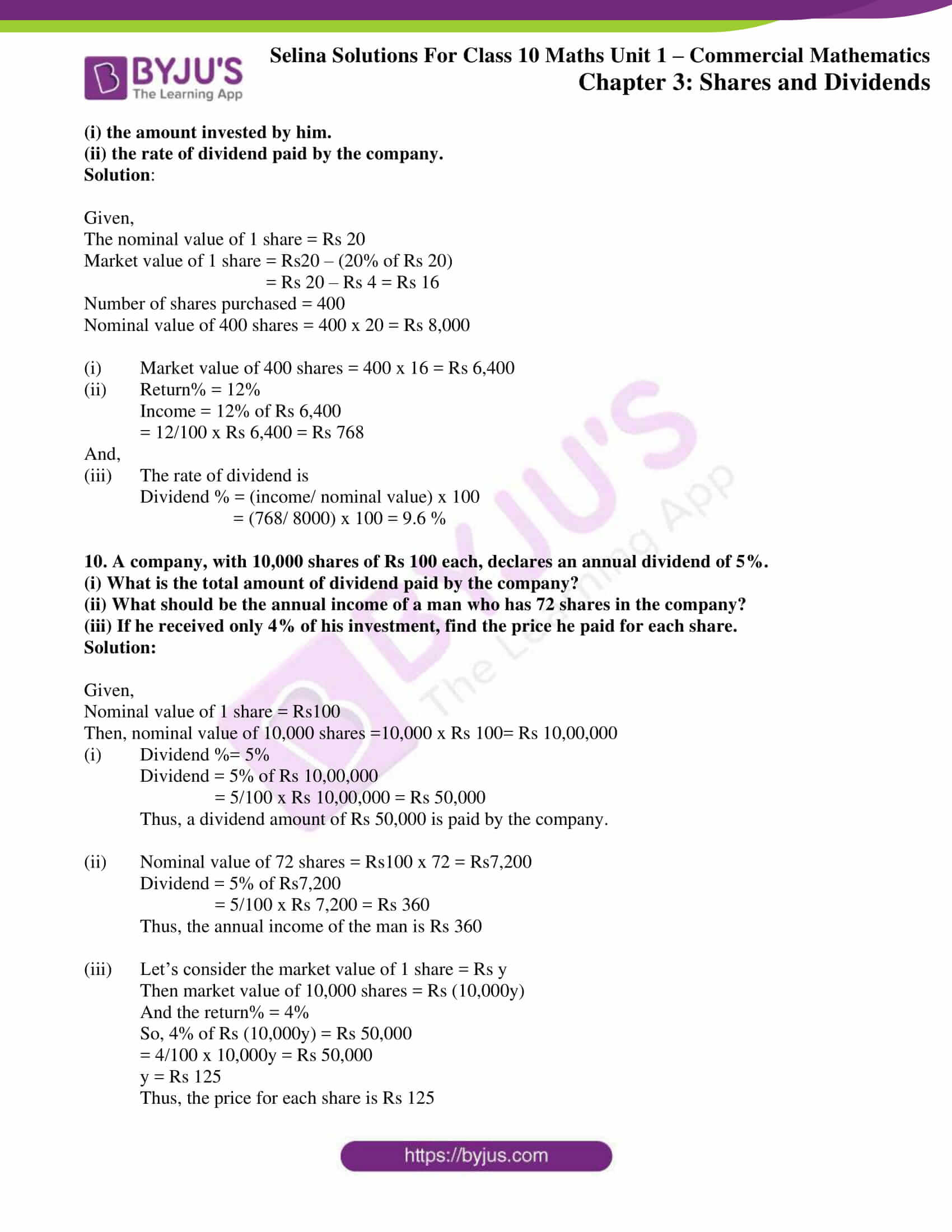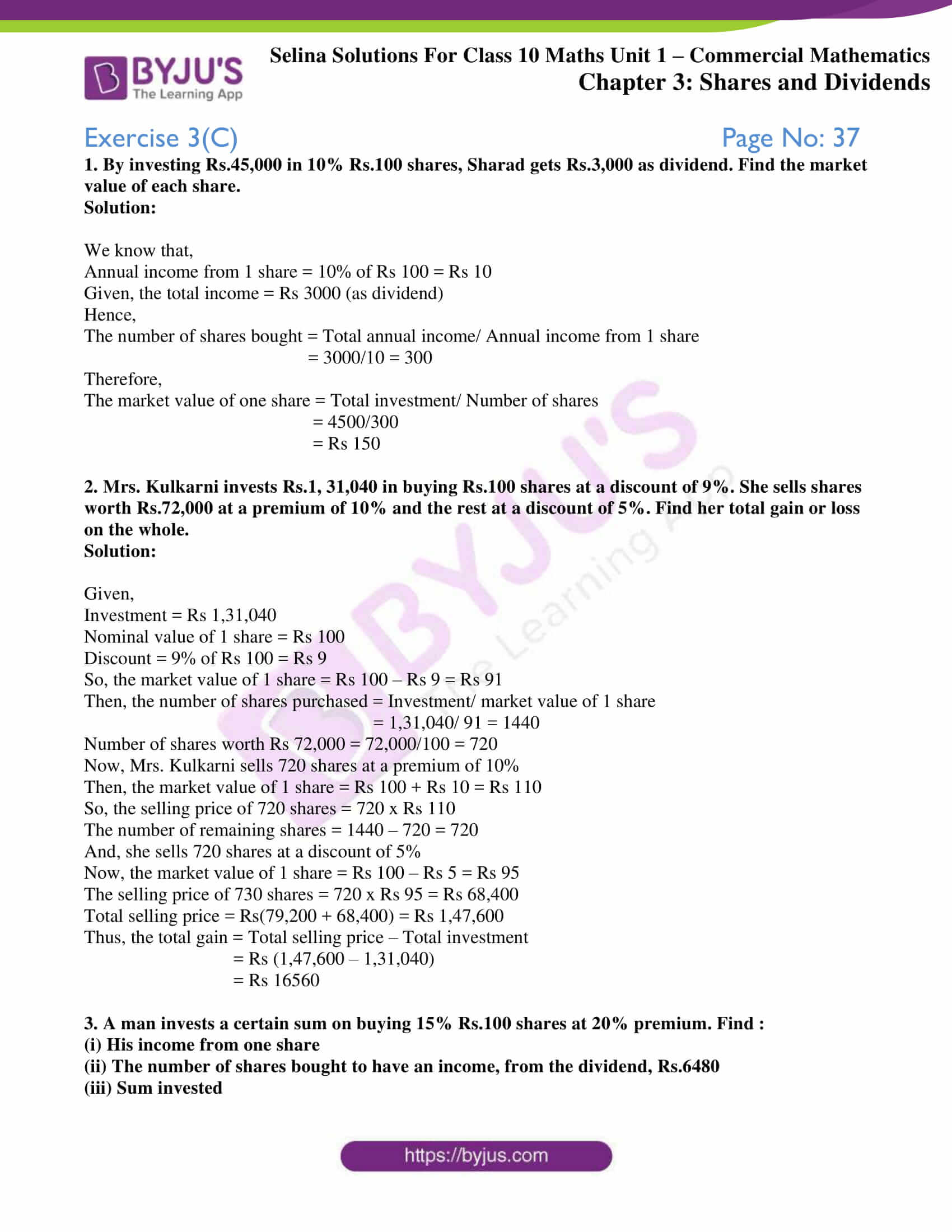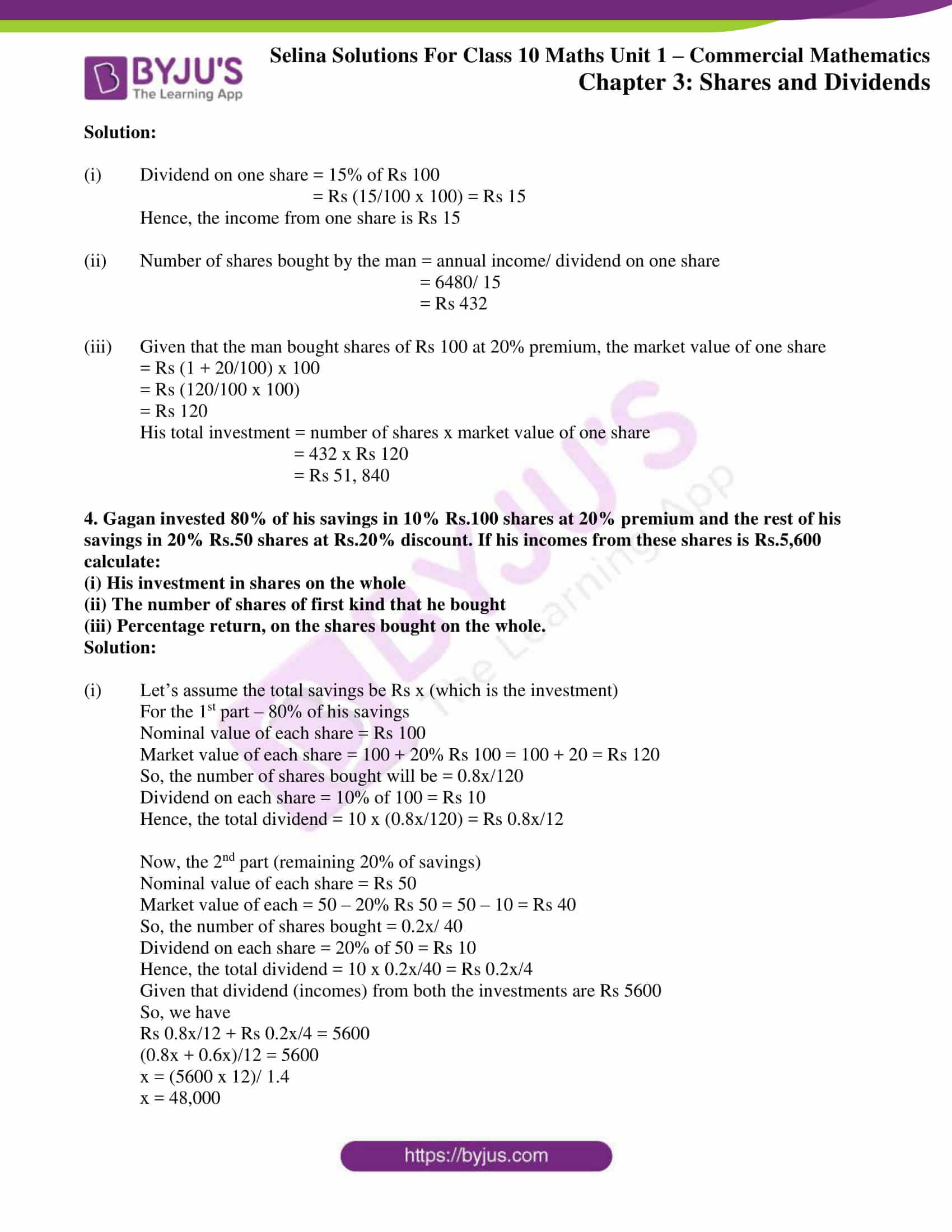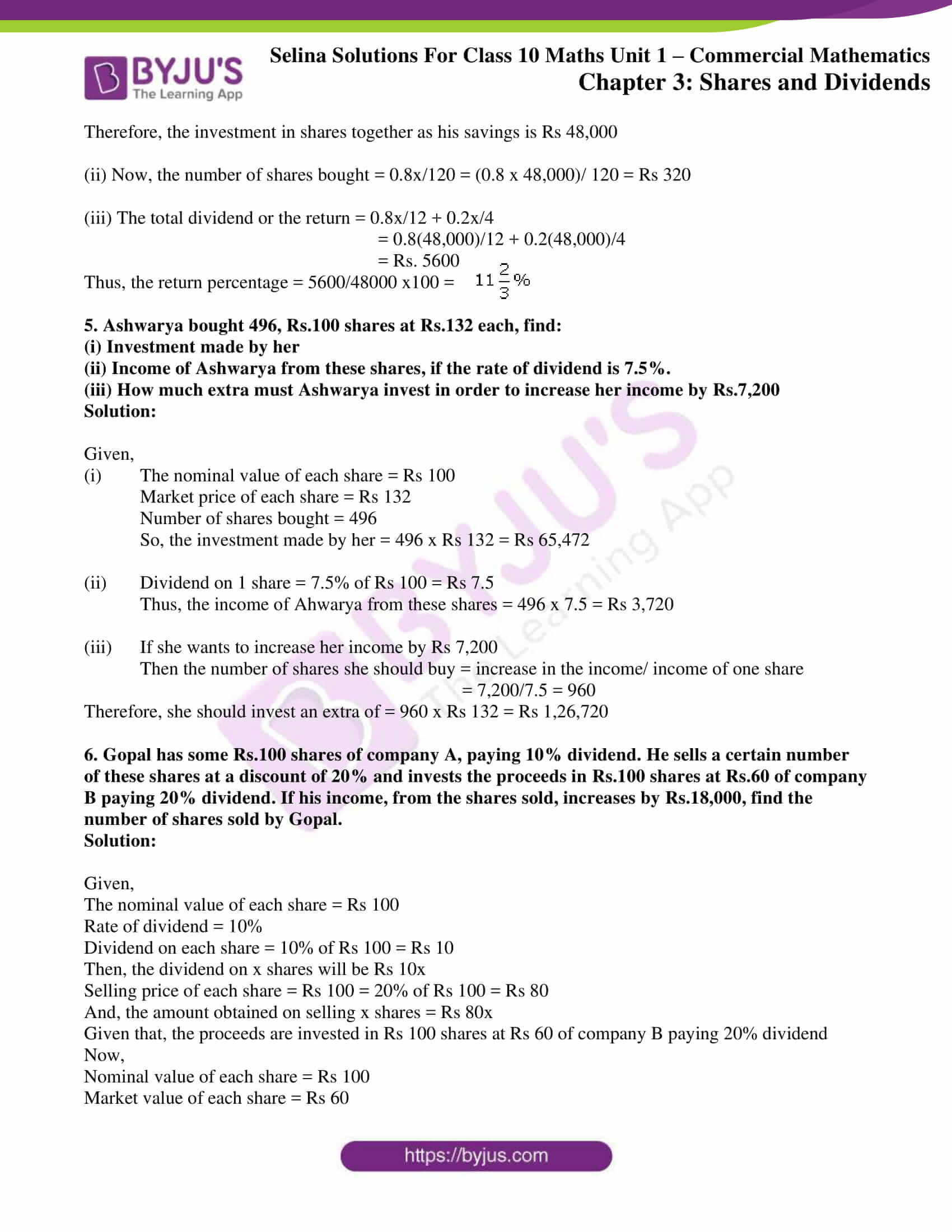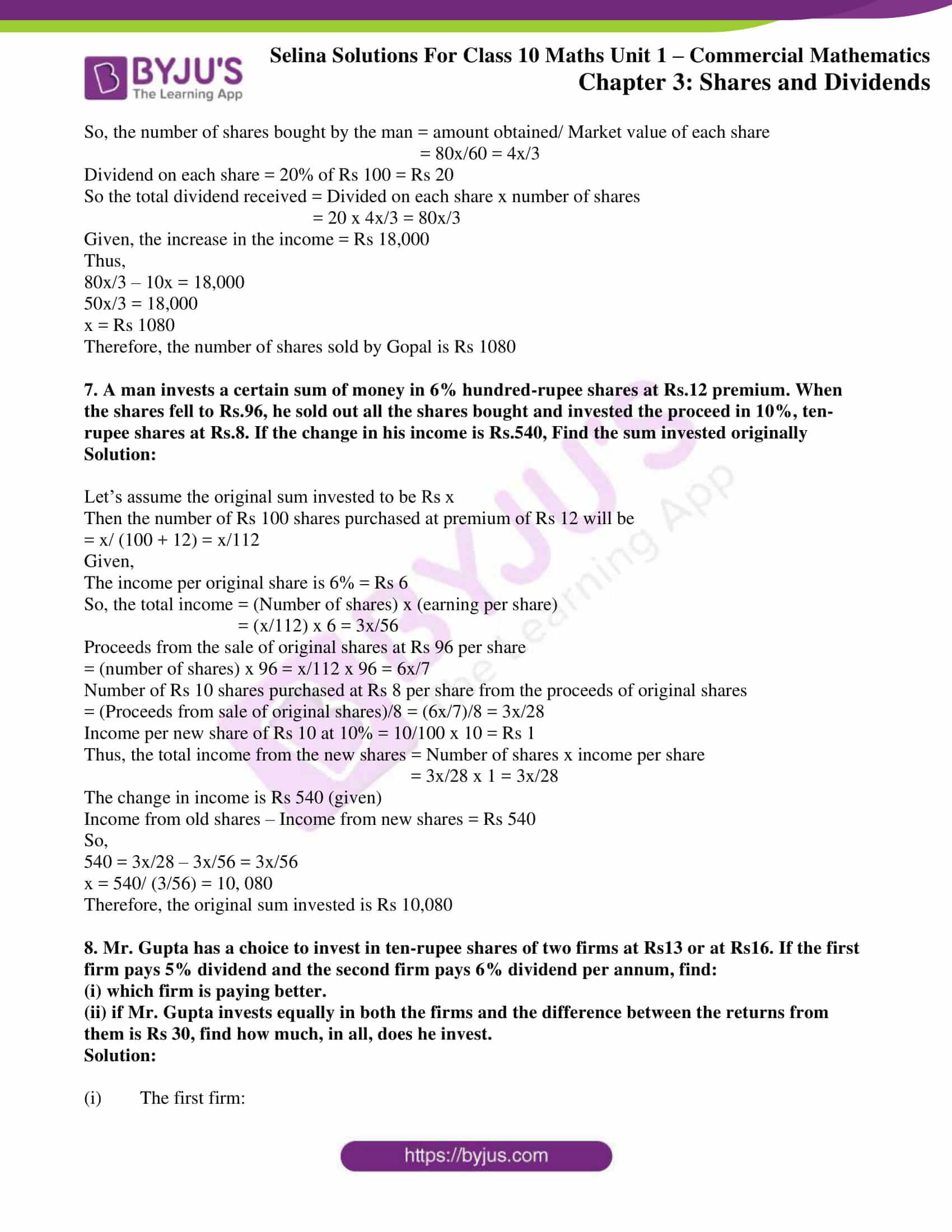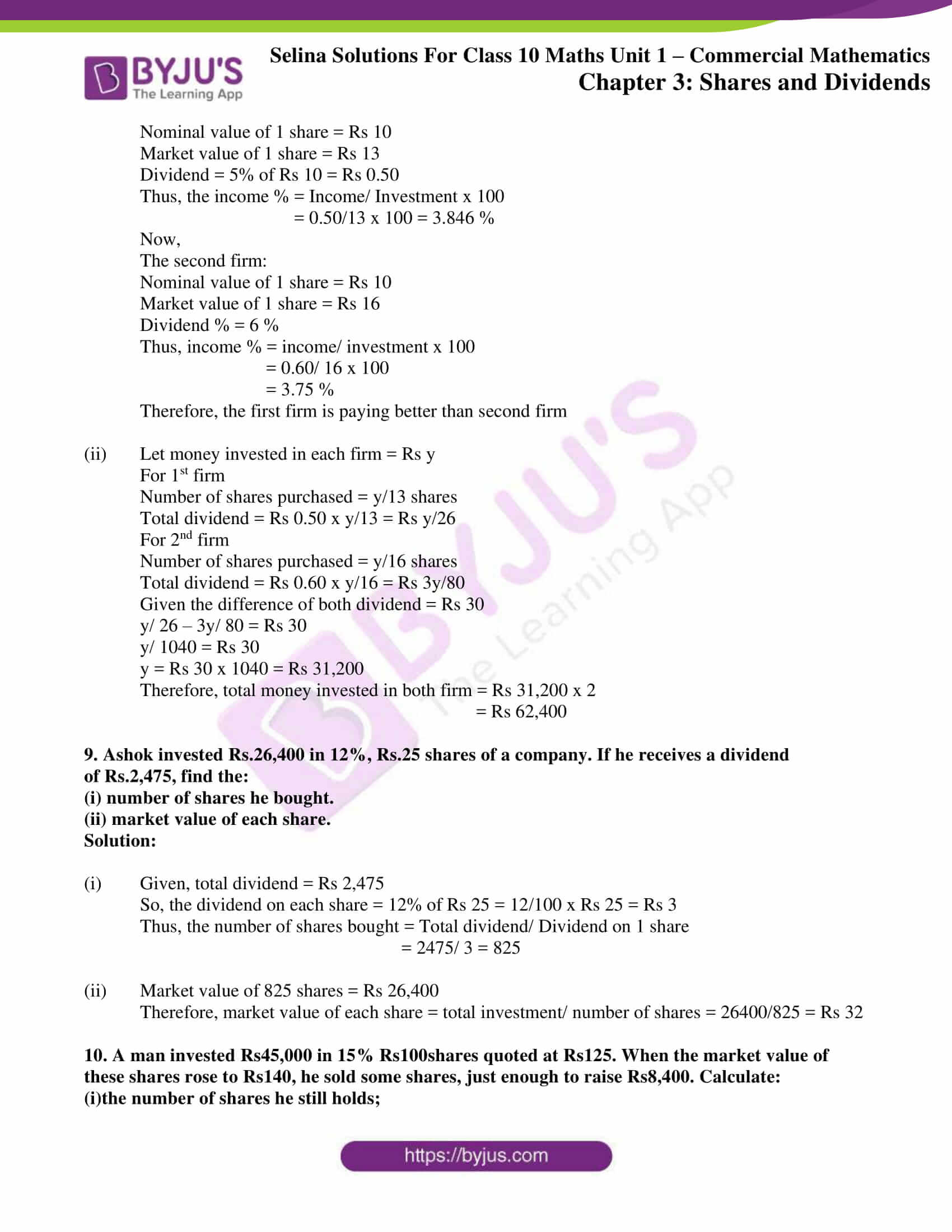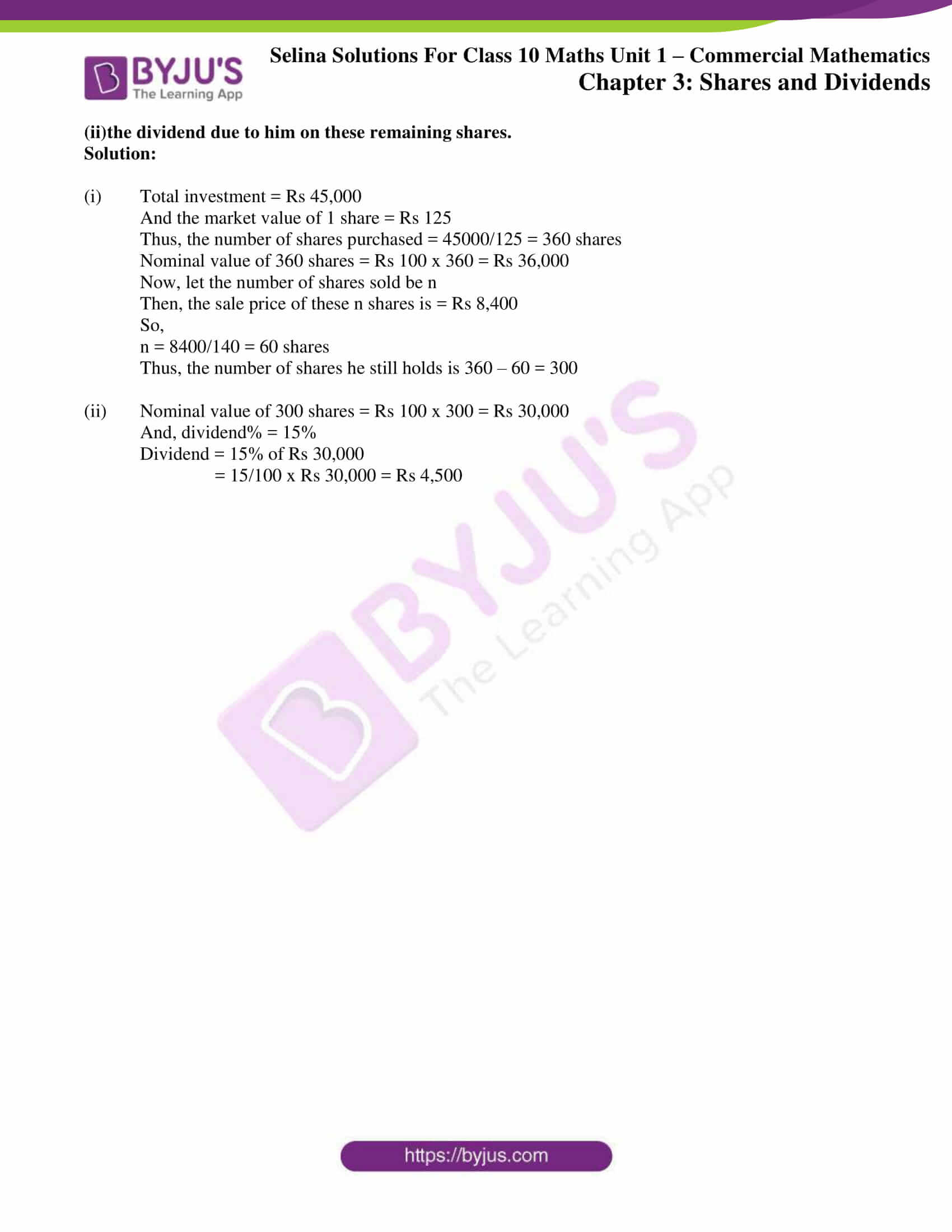### Exercises of Concise Selina Solutions Class 10 Maths Chapter 3 Shares and Dividends

Exercise 3(A) Solutions

Exercise 3(B) Solutions

Exercise 3(C) Solutions

## Access Selina Solutions Concise Maths Class 10 Chapter 3 Shares and Dividends

Exercise 3(A) Page No: 29

1. How much money will be required to buy 400, Rs.12.50 shares at a premium of Rs.1?

Solution:

Given,

The number of shares required to be bought = 400

And, Rs 12.50 shares at a premium of Rs 1 means;

Nominal value of the share is Rs. 12.50

And its market value = Rs 12.50 + Rs 1 = Rs 13.50

So, the money required to buy 1 share = Rs 13.50

Thus,

The money required to buy 400 shares = 400 x Rs 13.50 = Rs 5400

2. How much money will be required to buy 250, Rs.15 shares at a discount of Rs.1.50?

Solution:

The number of shares to be bought is 250.

And, Rs 15 shares at a discount of Rs 1.50 means

Nominal value of the share is Rs 15 and

Its market value = Rs 15 – Rs 1.50 = Rs 13.50

Thus,

The money required to buy 250 shares = 250 x Rs 13.50 = Rs 3375

3. A person buys 120 shares at a nominal value of Rs 40 each, which he sells at Rs 42.50 each. Find his profit and profit percent.

Solution:

Given,

The nominal value of each share is Rs 40

So, the nominal value of 120 shares = Rs 40 x 120 = Rs 4,800

And, the market value of 120 shares = Rs 42.50 × 120 = Rs 5,100

Thus, his profit = Rs 5,100 – Rs 4,800 = Rs 300

And the profit percentage is given by,

Profit (%) = 300/4800 x 100 = 6.25 %

4. Find the cost of 85 shares of Rs 60 each when quoted at Rs 63.25.

Solution:

Given,

Market value of 1 share = Rs 63.25

So, the market value of 85 shares = Rs 63.25 x 85 = Rs 5,376.25

5. A man invests Rs800 in buying Rs5 shares and when they are selling at a premium of Rs1.15, he sells all the shares. Find his profit and profit percent.

Solution:

Nominal value of 1 share = Rs 5

Market value 1 share = Rs 5 + Rs 1.15 = Rs6.15

Total money invested = Rs 800

So, the number of shares purchased = 800/5 = 160

And,

Market value of 160 shares = 160 x 6.15= Rs 984

Thus, his profit = Rs 984 – Rs 800 = Rs 184

And the profit percentage is given by

Profit (%) = 184/800 x 100 = 23 %

6. Find the annual income derived from 125, Rs.120 shares paying 5% dividend.

Solution:

Given,

The nominal value of 1 share = Rs 120

So, the nominal value of 125 shares = 125 x Rs 120 = Rs 15,000

Now,

Dividend = 5 % of Rs 15,000

⇒ 5/100 x 15000 = Rs 750

Thus, the annual income is Rs 750

7. A man invests Rs 3,072 in a company paying 5% per annum, when its Rs 10 share can be bought for Rs 16 each. Find:

(i) his annual income

(ii) his percentage income on his investment.

Solution:

Given,

Market value of 1 share = Rs 16

Nominal value of 1 share= Rs 10

And the money invested = Rs3,072

So, the number of shares purchased = 3072/16 = 192

And, the nominal value of 192 shares = Rs 10 x 192 = Rs 1,920

Therefore,

(i) The annual income = 5 % of Rs 1,920

= 5/100 x 1920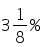= Rs 96

(ii) Income % = 96/3072 x 100 = 3.125% =

Exercise 3(B) Page No: 36

1. A man buys 75, Rs 100 shares paying 9 percent dividend. He buys shares at such a price that he gets 12 percent of his money. At what price did he buy the shares?

Solution:

Given,

Nominal value of 1 share = Rs100

So, the nominal value of 75 shares = 100 x 75 = Rs 7,500

And, Dividend % = 9 %

Thus, dividend = 9 % of Rs 7,500

= 9/100 x Rs 7,500 = Rs 675

Let’s consider the market price of 1 share = Rs y

Then the market price of 75 shares = Rs 75y

And, Profit % on investment = 12%

12% of 75y = Rs 657

12/100 x 75y = Rs 657

y = Rs 75

Therefore, the price of his shares is Rs 75 each

2. By purchasing Rs 25 gas shares for Rs 40 each, a man gets 4 percent profit on his investment. What rate percent is the company paying? What is his dividend if he buys 60 shares?

Solution:

Given,

Nominal value of 1 share = Rs25

Market value of 1 share = Rs40

And, the profit% on investment = 4%

Then profit on 1 share = 4% of Rs 40 = Rs 1.60

Thus,

Dividend % = 1.60/25 x 100 = 6.4%

Next,

If the number of shares purchased = 60

Then, the dividend on 60 shares = 60 x Rs 1.60 = Rs 96

3. Hundred rupee shares of a company are available in the market at a premium of Rs 20. Find the rate of dividend given by the company, when a man’s return on his investment is 15%.

Solution:

Given,

Nominal value of 1 share = Rs 100

And the market value of 1 share = Rs100 + Rs 20 = Rs120 (as the premium is Rs 20)

Also given, the profit % on investment of 1 share = 15%

Then profit = 15% of Rs 120 = Rs 18

Therefore,

Dividend % = 18/100 x 100 = 18%

4. Rs 50 shares of a company are quoted at a discount of 10%. Find the rate of dividend given by the company, the return on the investment on these shares being 20 percent.

Solution:

Given,

Nominal value of 1 share = Rs 50

Discount on each share = 10 %

So, the market value of 1 share = Rs50 – 10% of Rs50

= Rs 50 – Rs 5 = Rs 45

Also given, Profit % on investment = 20%

Then the profit on 1 share = 20% of Rs 45 = Rs 9

Therefore,

Dividend % = 9/50 x 100 = 18 %

5. A company declares 8 percent dividend to the shareholders. If a man receives Rs 2,840 as his dividend, find the nominal value of his shares.

Solution:

Given,

Dividend % = 8 %

And, the dividend is Rs 2,840

Let the nominal value of shares be Rs y

Then,

8% of y = Rs 2,840

(8/100) x y = Rs 2,840

y = Rs 35,500

Thus, the nominal value of the man’s share is Rs 35,500

6. How much should a man invest in Rs 100 shares selling at Rs 110 to obtain an annual income of Rs 1,680, if the dividend declared is 12%?

Solution:

From the question,

Nominal value of 1 share = Rs100

And, the market value of 1 share = Rs110

Let the number of shares purchased = n

Then the nominal value of n shares = Rs (100n)

Dividend % = 12%

Given that the dividend = Rs1,680

12 % of 100n = Rs 1,680

12/100 x 100n = Rs 1680So, the market value of 140 shares = 140 x 110 = Rs 15,400

Therefore the investment of the man should be Rs 15,400

7. A company declares a dividend of 11.2% to all its share-holders. If its Rs 60 share is available in the market at a premium of 25%, how much should Rakesh invest, in buying the shares of this company, in order to have an annual income of Rs 1,680?

Solution:

Given,

Nominal value of 1 share = Rs60

Market value of 1 share = Rs 60 + 25% of Rs 60

= Rs 60+ Rs 15= Rs 75

Let the number of shares purchased be n

Then, the nominal value of n shares = Rs (60n)

Dividend % = 11.2%

Given that the dividend = Rs 1,680

So, 11.2% of 60n = Rs 1,680

11.2/100 x 60n = Rs 1,680Then, the market value of 250 shares will be = 250 x 75 = Rs 18,750

Therefore, the investment of Rakesh should be Rs 18,750

8. A man buys 400, twenty-rupee shares at a premium of Rs 4 each and receives a dividend of 12%. Find:

(i) the amount invested by him.

(ii) his total income from the shares.

(iii) percentage return on his money.

Solution:

Given,

The nominal value of 1 share = Rs 20

Market value of 1 share = Rs 20 + Rs 4 = Rs 24

No. of shares purchased = 400

Nominal value of 400 shares = 400 x 20 = Rs 8,000

(i) Market value of 400 shares = 400 x 24 = Rs 9,600

(ii) Dividend% = 12%

Dividend = 12% of Rs 8,000

= 12/100 x Rs 8,000 = Rs 960

Thus, the total income from the shares is Rs 960

(iii) Percentage return on his money is

Return % = income/investment x 100

= (960/9600) x 100 = 10%

9. A man buys 400, twenty-rupee shares at a discount of 20% and receives a return of 12% on his money. Calculate:

(i) the amount invested by him.

(ii) the rate of dividend paid by the company.

Solution:

Given,

The nominal value of 1 share = Rs 20

Market value of 1 share = Rs20 – (20% of Rs 20)

= Rs 20 – Rs 4 = Rs 16

Number of shares purchased = 400

Nominal value of 400 shares = 400 x 20 = Rs 8,000

(i) Market value of 400 shares = 400 x 16 = Rs 6,400

(ii) Return% = 12%

Income = 12% of Rs 6,400

= 12/100 x Rs 6,400 = Rs 768

And,

(iii) The rate of dividend is

Dividend % = (income/ nominal value) x 100

= (768/ 8000) x 100 = 9.6 %

10. A company, with 10,000 shares of Rs 100 each, declares an annual dividend of 5%.

(i) What is the total amount of dividend paid by the company?

(ii) What should be the annual income of a man who has 72 shares in the company?

(iii) If he received only 4% of his investment, find the price he paid for each share.

Solution:

Given,

Nominal value of 1 share = Rs100

Then, nominal value of 10,000 shares =10,000 x Rs 100= Rs 10,00,000

(i) Dividend %= 5%

Dividend = 5% of Rs 10,00,000

= 5/100 x Rs 10,00,000 = Rs 50,000

Thus, a dividend amount of Rs 50,000 is paid by the company.

(ii) Nominal value of 72 shares = Rs100 x 72 = Rs7,200

Dividend = 5% of Rs7,200

= 5/100 x Rs 7,200 = Rs 360

Thus, the annual income of the man is Rs 360

(iii) Let’s consider the market value of 1 share = Rs y

Then market value of 10,000 shares = Rs (10,000y)

And the return% = 4%

So, 4% of Rs (10,000y) = Rs 50,000

= 4/100 x 10,000y = Rs 50,000

y = Rs 125

Thus, the price for each share is Rs 125

Exercise 3(C) Page No: 37

1. By investing Rs.45,000 in 10% Rs.100 shares, Sharad gets Rs.3,000 as dividend. Find the market value of each share.

Solution:

We know that,

Annual income from 1 share = 10% of Rs 100 = Rs 10

Given, the total income = Rs 3000 (as dividend)

Hence,

The number of shares bought = Total annual income/ Annual income from 1 share

= 3000/10 = 300

Therefore,

The market value of one share = Total investment/ Number of shares

= 4500/300

= Rs 150

2. Mrs. Kulkarni invests Rs.1, 31,040 in buying Rs.100 shares at a discount of 9%. She sells shares worth Rs.72,000 at a premium of 10% and the rest at a discount of 5%. Find her total gain or loss on the whole.

Solution:

Given,

Investment = Rs 1,31,040

Nominal value of 1 share = Rs 100

Discount = 9% of Rs 100 = Rs 9

So, the market value of 1 share = Rs 100 – Rs 9 = Rs 91

Then, the number of shares purchased = Investment/ market value of 1 share

= 1,31,040/ 91 = 1440

Number of shares worth Rs 72,000 = 72,000/100 = 720

Now, Mrs. Kulkarni sells 720 shares at a premium of 10%

Then, the market value of 1 share = Rs 100 + Rs 10 = Rs 110

So, the selling price of 720 shares = 720 x Rs 110

The number of remaining shares = 1440 – 720 = 720

And, she sells 720 shares at a discount of 5%

Now, the market value of 1 share = Rs 100 – Rs 5 = Rs 95

The selling price of 730 shares = 720 x Rs 95 = Rs 68,400

Total selling price = Rs(79,200 + 68,400) = Rs 1,47,600

Thus, the total gain = Total selling price – Total investment

= Rs (1,47,600 – 1,31,040)

= Rs 16560

3. A man invests a certain sum on buying 15% Rs.100 shares at 20% premium. Find :

(i) His income from one share

(ii) The number of shares bought to have an income, from the dividend, Rs.6480

(iii) Sum invested

Solution:

(i) Dividend on one share = 15% of Rs 100

= Rs (15/100 x 100) = Rs 15

Hence, the income from one share is Rs 15

(ii) Number of shares bought by the man = annual income/ dividend on one share

= 6480/ 15

= Rs 432

(iii) Given that the man bought shares of Rs 100 at 20% premium, the market value of one share

= Rs (1 + 20/100) x 100

= Rs (120/100 x 100)

= Rs 120

His total investment = number of shares x market value of one share

= 432 x Rs 120

= Rs 51, 840

4. Gagan invested 80% of his savings in 10% Rs.100 shares at 20% premium and the rest of his savings in 20% Rs.50 shares at Rs.20% discount. If his incomes from these shares is Rs.5,600 calculate:

(i) His investment in shares on the whole

(ii) The number of shares of first kind that he bought

(iii) Percentage return, on the shares bought on the whole.

Solution:

(i) Let’s assume the total savings be Rs x (which is the investment)

For the 1st part – 80% of his savings

Nominal value of each share = Rs 100

Market value of each share = 100 + 20% Rs 100 = 100 + 20 = Rs 120

So, the number of shares bought will be = 0.8x/120

Dividend on each share = 10% of 100 = Rs 10

Hence, the total dividend = 10 x (0.8x/120) = Rs 0.8x/12

Now, the 2nd part (remaining 20% of savings)

Nominal value of each share = Rs 50

Market value of each = 50 – 20% Rs 50 = 50 – 10 = Rs 40

So, the number of shares bought = 0.2x/ 40

Dividend on each share = 20% of 50 = Rs 10

Hence, the total dividend = 10 x 0.2x/40 = Rs 0.2x/4

Given that dividend (incomes) from both the investments are Rs 5600

So, we have

Rs 0.8x/12 + Rs 0.2x/4 = 5600

(0.8x + 0.6x)/12 = 5600

x = (5600 x 12)/ 1.4

x = 48,000

Therefore, the investment in shares together as his savings is Rs 48,000

(ii) Now, the number of shares bought = 0.8x/120 = (0.8 x 48,000)/ 120 = Rs 320

(iii) The total dividend or the return = 0.8x/12 + 0.2x/4

= 0.8(48,000)/12 + 0.2(48,000)/4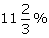= Rs. 5600

Thus, the return percentage = 5600/48000 x100 =

5. Ashwarya bought 496, Rs.100 shares at Rs.132 each, find:

(ii) Income of Ashwarya from these shares, if the rate of dividend is 7.5%.

(iii) How much extra must Ashwarya invest in order to increase her income by Rs.7,200

Solution:

Given,

(i) The nominal value of each share = Rs 100

Market price of each share = Rs 132

Number of shares bought = 496

So, the investment made by her = 496 x Rs 132 = Rs 65,472

(ii) Dividend on 1 share = 7.5% of Rs 100 = Rs 7.5

Thus, the income of Ahwarya from these shares = 496 x 7.5 = Rs 3,720

(iii) If she wants to increase her income by Rs 7,200

Then the number of shares she should buy = increase in the income/ income of one share

= 7,200/7.5 = 960

Therefore, she should invest an extra of = 960 x Rs 132 = Rs 1,26,720

6. Gopal has some Rs.100 shares of company A, paying 10% dividend. He sells a certain number of these shares at a discount of 20% and invests the proceeds in Rs.100 shares at Rs.60 of company B paying 20% dividend. If his income, from the shares sold, increases by Rs.18,000, find the number of shares sold by Gopal.

Solution:

Given,

The nominal value of each share = Rs 100

Rate of dividend = 10%

Dividend on each share = 10% of Rs 100 = Rs 10

Then, the dividend on x shares will be Rs 10x

Selling price of each share = Rs 100 = 20% of Rs 100 = Rs 80

And, the amount obtained on selling x shares = Rs 80x

Given that, the proceeds are invested in Rs 100 shares at Rs 60 of company B paying 20% dividend

Now,

Nominal value of each share = Rs 100

Market value of each share = Rs 60

So, the number of shares bought by the man = amount obtained/ Market value of each share

= 80x/60 = 4x/3

Dividend on each share = 20% of Rs 100 = Rs 20

So the total dividend received = Divided on each share x number of shares

= 20 x 4x/3 = 80x/3

Given, the increase in the income = Rs 18,000

Thus,

80x/3 – 10x = 18,000

50x/3 = 18,000

x = Rs 1080

Therefore, the number of shares sold by Gopal is Rs 1080

7. A man invests a certain sum of money in 6% hundred-rupee shares at Rs.12 premium. When the shares fell to Rs.96, he sold out all the shares bought and invested the proceed in 10%, ten-rupee shares at Rs.8. If the change in his income is Rs.540, Find the sum invested originally

Solution:

Let’s assume the original sum invested to be Rs x

Then the number of Rs 100 shares purchased at premium of Rs 12 will be

= x/ (100 + 12) = x/112

Given,

The income per original share is 6% = Rs 6

So, the total income = (Number of shares) x (earning per share)

= (x/112) x 6 = 3x/56

Proceeds from the sale of original shares at Rs 96 per share

= (number of shares) x 96 = x/112 x 96 = 6x/7

Number of Rs 10 shares purchased at Rs 8 per share from the proceeds of original shares

= (Proceeds from sale of original shares)/8 = (6x/7)/8 = 3x/28

Income per new share of Rs 10 at 10% = 10/100 x 10 = Rs 1

Thus, the total income from the new shares = Number of shares x income per share

= 3x/28 x 1 = 3x/28

The change in income is Rs 540 (given)

Income from old shares – Income from new shares = Rs 540

So,

540 = 3x/28 – 3x/56 = 3x/56

x = 540/ (3/56) = 10, 080

Therefore, the original sum invested is Rs 10,080

8. Mr. Gupta has a choice to invest in ten-rupee shares of two firms at Rs13 or at Rs16. If the first firm pays 5% dividend and the second firm pays 6% dividend per annum, find:

(i) which firm is paying better.

(ii) if Mr. Gupta invests equally in both the firms and the difference between the returns from them is Rs 30, find how much, in all, does he invest.

Solution:

(i) The first firm:

Nominal value of 1 share = Rs 10

Market value of 1 share = Rs 13

Dividend = 5% of Rs 10 = Rs 0.50

Thus, the income % = Income/ Investment x 100

= 0.50/13 x 100 = 3.846 %

Now,

The second firm:

Nominal value of 1 share = Rs 10

Market value of 1 share = Rs 16

Dividend % = 6 %

Thus, income % = income/ investment x 100

= 0.60/ 16 x 100

= 3.75 %

Therefore, the first firm is paying better than second firm

(ii) Let money invested in each firm = Rs y

For 1st firm

Number of shares purchased = y/13 shares

Total dividend = Rs 0.50 x y/13 = Rs y/26

For 2nd firm

Number of shares purchased = y/16 shares

Total dividend = Rs 0.60 x y/16 = Rs 3y/80

Given the difference of both dividend = Rs 30

y/ 26 – 3y/ 80 = Rs 30

y/ 1040 = Rs 30

y = Rs 30 x 1040 = Rs 31,200

Therefore, total money invested in both firm = Rs 31,200 x 2

= Rs 62,400

9. Ashok invested Rs.26,400 in 12%, Rs.25 shares of a company. If he receives a dividend of Rs.2,475, find the:

(i) number of shares he bought.

(ii) market value of each share.

Solution:

(i) Given, total dividend = Rs 2,475

So, the dividend on each share = 12% of Rs 25 = 12/100 x Rs 25 = Rs 3

Thus, the number of shares bought = Total dividend/ Dividend on 1 share

= 2475/ 3 = 825

(ii) Market value of 825 shares = Rs 26,400

Therefore, market value of each share = total investment/ number of shares = 26400/825 = Rs 32

10. A man invested Rs45,000 in 15% Rs100shares quoted at Rs125. When the market value of these shares rose to Rs140, he sold some shares, just enough to raise Rs8,400. Calculate:

(i)the number of shares he still holds;

(ii)the dividend due to him on these remaining shares.

Solution:

(i) Total investment = Rs 45,000

And the market value of 1 share = Rs 125

Thus, the number of shares purchased = 45000/125 = 360 shares

Nominal value of 360 shares = Rs 100 x 360 = Rs 36,000

Now, let the number of shares sold be n

Then, the sale price of these n shares is = Rs 8,400

So,

n = 8400/140 = 60 shares

Thus, the number of shares he still holds is 360 – 60 = 300

(ii) Nominal value of 300 shares = Rs 100 x 300 = Rs 30,000

And, dividend% = 15%

Dividend = 15% of Rs 30,000

= 15/100 x Rs 30,000 = Rs 4,500

The given solutions are as per the 2019-20 Concise Selina textbook. The Selina Solutions for the academic year 2020-21 will be updated soon.